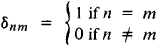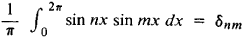# Kronecker Delta

Also found in: Dictionary, Thesaurus, Wikipedia.

## Kronecker delta

[′krō·nek·ər ‚del·tə]
(mathematics)
The function or symbol δij dependent upon the subscripts i and j which are usually integers; its value is 1 if i = j and 0 if ij.
McGraw-Hill Dictionary of Scientific & Technical Terms, 6E, Copyright © 2003 by The McGraw-Hill Companies, Inc.
The following article is from The Great Soviet Encyclopedia (1979). It might be outdated or ideologically biased.

## Kronecker Delta

a function δnm that is dependent on two integral arguments n and m and is defined byAn example of the use of the Kronecker symbol isThe Kronecker symbol was introduced by L. Kronecker in 1866.

The Great Soviet Encyclopedia, 3rd Edition (1970-1979). © 2010 The Gale Group, Inc. All rights reserved.
Mentioned in ?
References in periodicals archive ?
The number of separate terms in the expressions for the generalized Kronecker delta, if written only by using the standard Kronecker delta symbol, is given by the so-called double factorial (2n)!/(2"n!).
Lemma 3.3 Let [[alpha].sub.i] = [C.sub.i-1] + [[delta].sub.i,1] where [[delta].sub.i,1] denotes the Kronecker delta. Then the central binomial coefficient is given by the sum
where [R.sub.out] = R(x, [y.sub.obs], z), [R.sub.in] = R(x, -[y.sub.obs], z), [[delta].sub.wg] is the Kronecker delta, g = [perpendicular to], [parallel] and w = [perpendicular to], [parallel] represent the incident and scattered wave polarization, respectively, and
where [MATHEMATICAL EXPRESSION NOT REPRODUCIBLE IN ASCII] denotes system (1)-(2) response to Kronecker delta [delta](k - [k.sub.0]) at time [k.sub.1], i.e.
Let [[delta].sub.i] (j) be the Kronecker delta function on S, that is for i, j [member] S
[[delta].sub.ij] is the Kronecker delta tensor and [eta] is the viscosity.
where [[delta].sub.k-j] is the Kronecker delta function given by
where the brackets <> denote the so-called projection, i.e., the inner product and where [delta] denotes the Kronecker delta. From these basis vectors, we define their tetrad components as
where the Kronecker delta guarantees the neutrality of the system.
with I = 1/2([[delta].sub.ik][[delta].sub.jl] + [[delta].sub.il][[delta].sub.jk]), where [delta] is the Kronecker delta. Finally, the continuum tangent elastoplastic tensor [a.sup.ep] is defined as

Site: Follow: Share:
Open / Close